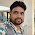Sample Questions, Previous Year Solved Papers, Study Materials For Competitive Examinations Like UGC NET, SET And GATE Computer Science.

## Wednesday, 15 April 2015

1.       The following deterministic finite automata recognizes:
(A) Set of all strings containing ‘ab’
(B) Set of all strings containing ‘aab’
(C) Set of all strings ending in ‘abab’
(D) None of the above
2.       Depth ion travels of the following directed graph is:
(A) ABCDEF
(B) ABDEFC
(C) ACEBDF
(D) None of the above
3.       The maximum number of nodes in a binary tree of depth 10 is:
(A) 1024
(B) 210-1
(C) 1000
(D) None of the above
4.       The regular expression given below describes:
r=(1+01)*(0+λ)
(A) Set of all string not containing ‘11’
(B) Set of all string not containing ‘00’
(C) Set of all string containing ‘01’
(D) Set of all string ending in ‘0’
5.       Which of the following language is regular?
(A) L={anbn|n≥1}
(B) L={anbmcndm|n,m≥1}
(C) L={anbm|n,m≥1}
(D) L={anbmcn|n,m≥1}

6.       2’s complement of -100 is:
(A) 00011100
(B) 10011101
(C) 10011100
(D) 11100100
7.       Which of the following expression remove hazard form: xy+zx’ ?
(A) xy+zx’
(B) xy+zx’
(C) xy+zx’+yz
(D) xy+zx’+wz
8.       How many 1’s are present in the binary representation of 15x256+5x16+3:
(A) 8
(B) 9
(C) 10
(D) 11
9.       If AB=C, then:
(A) AC=B
(B) BC=A
(C) ABC=1
(D) ABC=0
10.    What is the maximum counting speed of a 4-bit binary counter which is composed of Flip-Flop with a propagation delay of 25ns?
(A) 1MHz
(B) 10MHz
(C) 100MHz
(D) 4MHz

Pages   2   3   4   5

1.2.